# 双重循环嵌套执行原理(逆战班)

<script>
/* 只能被1和它自身除尽的数称为质数 */
let sum = 0;
// 质数是一个大于1的自然数,所以定义的变量i=2,从2开始循环
// 并且这里只计算100以内的质数,所以循环到100的时候就跳出
for (let i = 2; i < 100; i++) {
// 定义一个变量s,当每次循环结果是质数或非质数,输出不同的s值
let s = 1;
// 定义一个变量j,并且j的值要一直小于i
for (let j = 2; j < i; j++) {
// 上面说的要j的值一直小于i.目的就是为了让i除以j来取余
if (i % j === 0) {
// 当i除以j取余为零,s输出结果就赋值为0.也就是除尽了,不是质数
s = 0;
// 跳出当前循环,会重新定义s=1.
break;
}
}
// 这里s===1也就意味着前面没除尽的数,没有对s进行改变,所以s为原来的初始状态.
// 也就是质数
if (s === 1) {
console.log(i + '这是质数');
// 将最先定义的一个为0的变量不断累加i的值,得到的就是质数的和。
sum += i;
}

if (s === 0) {
console.log(i + '这不是质数');
}
}
// 最后输出质数的总和。
console.log(sum);
</script>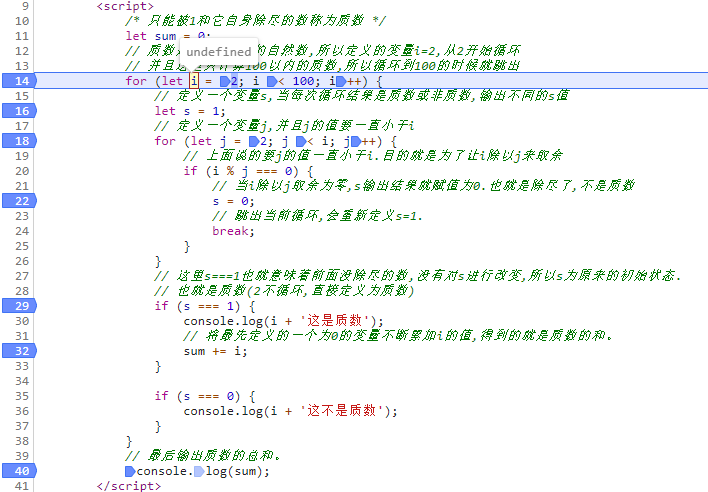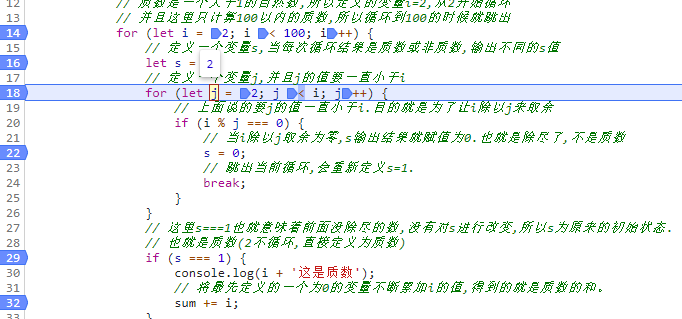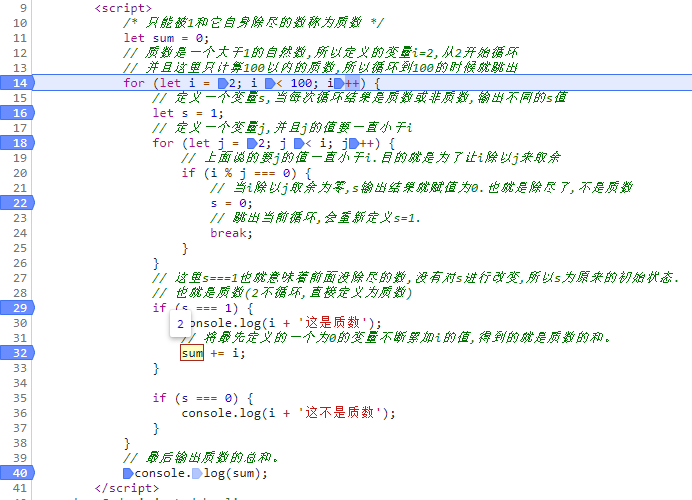if (s === 1) {
console.log(i + '这是质数');
// 将最先定义的一个为0的变量不断累加i的值,得到的就是质数的和。
sum += i;
}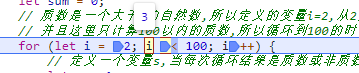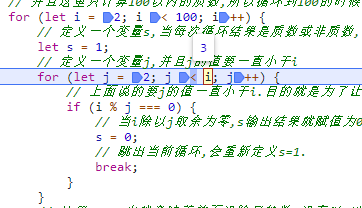for (let j = 2; j < i; j++)


for (let j = 2; 2 < 4; j++)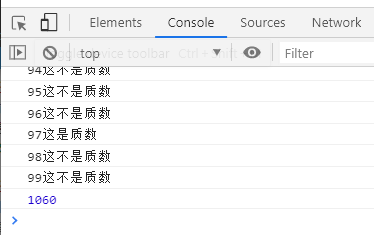01-21365503-2158
03-221402
03-2237
03-21738
03-14959
03-16159
03-1441
03-2333
03-14324
05-06
04-301万+